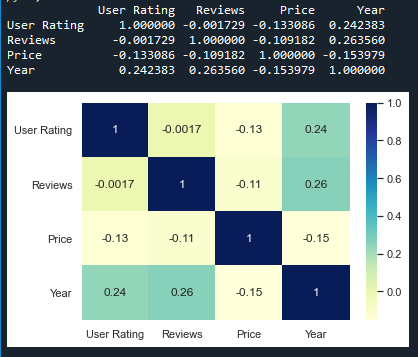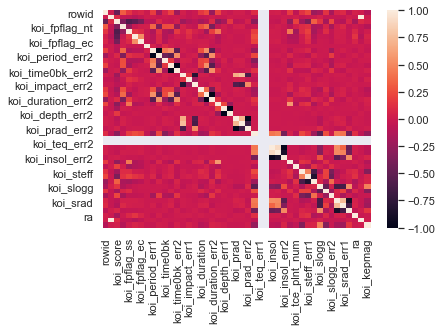Related Articles
How to create a seaborn correlation heatmap in Python?
• Last Updated : 12 Nov, 2020

Seaborn is a Python library that is based on matplotlib and is used for data visualization. It provides a medium to present data in a statistical graph format as an informative and attractive medium to impart some information. A heatmap is one of the components supported by seaborn where variation in related data is portrayed using a color palette. This article centrally focuses on a correlation heatmap and how seaborn in combination with pandas and matplotlib can be used to generate one for a dataframe.

### Installation

Like any another Python library, seaborn can be easily installed using pip:

```pip install seaborn
```

This library is a part of Anaconda distribution and usually works just by import if your IDE is supported by Anaconda, but it can be installed too by the following command:

```conda install seaborn
```

## Correlation heatmap

A correlation heatmap is a heatmap that shows a 2D correlation matrix between two discrete dimensions, using colored cells to represent data from usually a monochromatic scale. The values of the first dimension appear as the rows of the table while of the second dimension as a column. The color of the cell is proportional to the number of measurements that match the dimensional value. This makes correlation heatmaps ideal for data analysis since it makes patterns easily readable and highlights the differences and variation in the same data. A correlation heatmap, like a regular heatmap, is assisted by a colorbar making data easily readable and comprehensible.

The following steps show how a correlation heatmap can be produced:

• Import all required modules first
• Import the file where your data is stored
• Plot a heatmap
• Display it using matplotlib

For plotting heatmap method of the seaborn module will be used.

Syntax: heatmap(data, vmin, vmax, center, cmap,……………………………………………………)

Except for data all other attributes are optional and data obviously will be the data to be plotted. The data here has to be passed with corr() method to generate a correlation heatmap. Also, corr() itself eliminates columns which will be of no use while generating a correlation heatmap and selects those which can be used.

Example 1:

For the example given below, here a dataset downloaded from kaggle.com is being used. The plot shows data related to bestseller novels on amazon.

Dataset used – Bestsellers

## Python3

 `# import modules``import` `matplotlib.pyplot as mp``import` `pandas as pd``import` `seaborn as sb`` ` `# import file with data``data ``=` `pd.read_csv(``"C:\\Users\\Vanshi\\Desktop\\bestsellers.csv"``)`` ` `# prints data that will be plotted``# columns shown here are selected by corr() since``# they are ideal for the plot``print``(data.corr())`` ` `# plotting correlation heatmap``dataplot ``=` `sb.heatmap(data.corr(), cmap``=``"YlGnBu"``, annot``=``True``)`` ` `# displaying heatmap``mp.show()`

Output:The above example deals with small data. The following example depicts how the output will look like for a large dataset,

Example 2:

The dataset used in this example is an exoplanet space research dataset compiled by NASA.

Dataset used – cumulative

## Python3

 `# import modules``import` `matplotlib.pyplot as mp``import` `pandas as pd``import` `seaborn as sb`` ` `# import file with data``data``=``pd.read_csv(``"C:\\Users\\Vanshi\\Desktop\\cumulative.csv"``)`` ` `# plotting correlation heatmap``dataplot``=``sb.heatmap(data.corr())`` ` `# displaying heatmap``mp.show()`

Output:Attention geek! Strengthen your foundations with the Python Programming Foundation Course and learn the basics.

To begin with, your interview preparations Enhance your Data Structures concepts with the Python DS Course.

My Personal Notes arrow_drop_up